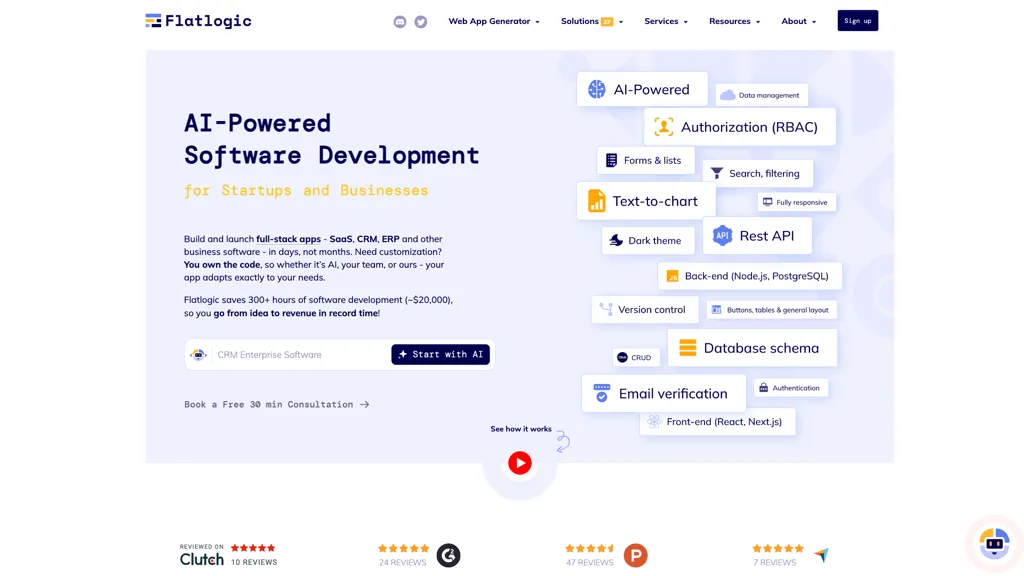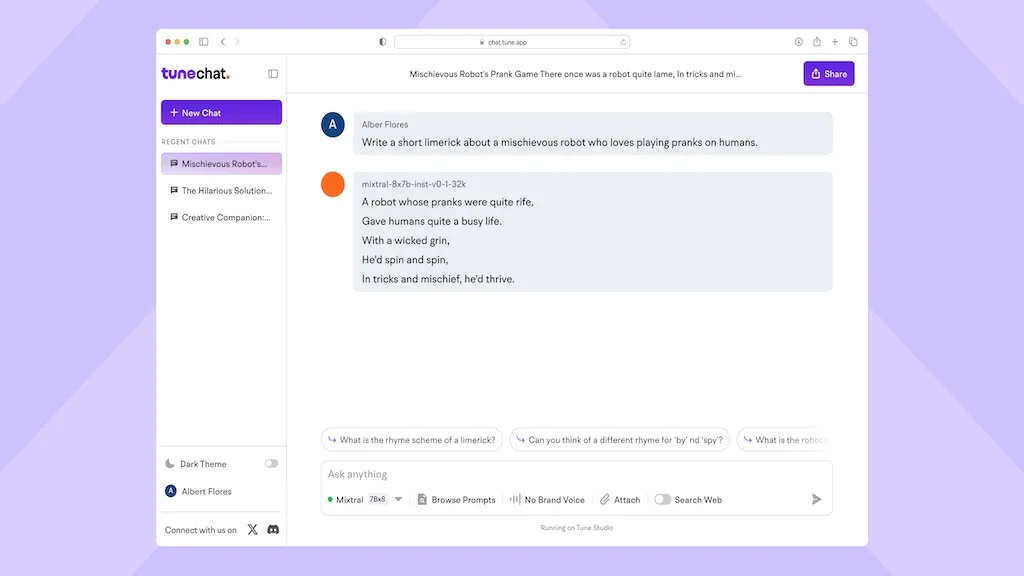# Interactive Mathematics

Save

## What is Interactive Mathematics? 5 0 ratings

The AI math problem solver is an interactive mathematics tool that offers step-by-step solutions to math homework problems.It combines a powerful mathematical computational engine with an artificial intelligence language model to provide accurate solutions.

It can handle a wide range of math problems, from tough word problems to algebra equations and advanced calculus.The tool also offers math tutoring through a chat platform.Users can sign up and submit their math problems to receive personalized solutions.

Additionally, it provides free math lessons to help students improve their math skills.The AI math problem solver can interpret math word problems and determine the mathematical operations required to solve them.

Interactive Mathematics Details

Pricing: Free Check Pricing Page

Tagged: Education Students

🔥 Promote this tool

## Interactive Mathematics possible use cases:

1. Learn how to solve math problems.
2. Practice your math problems and improve skills.
3. Learn math interactively with the help of an AI tutor.##### Related Searches
math problem solver math homework solver ai math tutor
Share it:
##### How do you rate Interactive Mathematics?

5 0 ratings

Breakdown 👇

Interactive Mathematics is not rated yet, be the first to rate it

#### Featured Tools 🌟##### Flatlogic##### GPTGO##### Wonderchat##### interview.study##### Blainy##### WebWave AI##### Chatsimple - AI Chatbot##### Recall##### Vellum##### Octopus.do##### ChatNBX